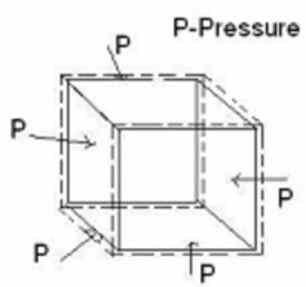Bulk Modulus Calculator

# Bulk Modulus Calculation

The calculator will calculate the bulk modulus or pressure or initial volume or change in volume as per selection from drop-down and given appropriate values. The Bulk Modulus is defined as the pressure increase needed to cause a given relative decrease in volume.
Bulk Modulus: B = P x Vi/Vc; Pressure: P = B x Vc/Vi; Initial Volume: Vi = B x Vc/P; Change in Volume: Vc = P x Vi/B
where, B = Bulk Modulus. p = Pressure, Vi = Initial Volume, Vc = Change in Volume.

 I want to calculate Bulk Modulus(B) Pressure(p) Initial Volume(Vi) Change in Volume(Vc) Pressure(p) = P Initial Volume(Vi) = m3 Change in Volume(Vc) = m3 Bulk Modulus(B) = N/m2
 The bulk modulus(B) is a measure of the ability of a substance to withstand volume changes under compression on all sides. The Bulk modulus is a numerical constant that describes the elastic properties of any solid or fluid when it is under pressure from all surfaces. The pressure applied reduces the volume of the material and when pressure is removed the material returns to its original volume. This is referred to as the incompressibility. It is equal to the quotient of the applied pressure divided by the relative deformation. The relative deformation also called as strain. The strain is the change in volume divided by the initial volume. If initial volume Vi of a material is reduced by an applied pressure P to a new volume Vn, the strain may be expressed as the change in volume (Vc) equals to Vi − Vn, divided by the initial volume, or Vc/Vi.